# Tamil Nadu 11th Chemistry Model Question Paper 3 English Medium

Students can Download Tamil Nadu 11th Chemistry Model Question Paper 3 English Medium Pdf, Tamil Nadu 11th Chemistry Model Question Papers helps you to revise the complete Tamilnadu State Board New Syllabus and score more marks in your examinations.

## TN State Board 11th Chemistry Model Question Paper 3 English MediumTime: 2½ Hours
Total Score: 70 Marks

General Instructions:

1. The question paper comprises of four parts.
2. You are to attempt all the parts. An internal choice of questions is provided wherever applicable.
3. All questions of Part I. II, III and IV are to be attempted separately.
4. Question numbers 1 to 15 in Part I are Multiple Choice Questions of one mark each. These are to be answered by choosing the most suitable answer from the given four alternatives and writing the option code and the corresponding answer.
5. Question numbers 16 to 24 in Part II are two-mark questions. These are to be answered in about one or two sentences.
6. Question numbers 25 to 33 in Part III are three-mark questions. These are to be answered in about three to five short sentences.
7. Question numbers 34 to 38 in Part IV are five-mark questions. These are to be answered in detail. Draw diagrams wherever necessary.

Part – I

Choose the most suitable answer from the given four alternatives.

Question 1.
Choose the disproportionation reaction among the following redox reactions.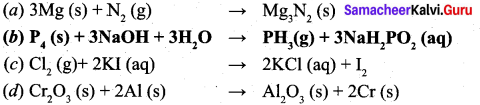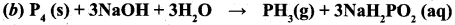Solution:
$$P_{4}(s)+3 N a O H+3 H_{2} \rightarrow P H_{3}(g)+3 N a H_{2} P O_{2}(a q)$$

Question 2.
Consider the following sets of quantum numbers: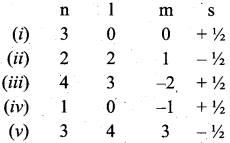Which of the following sets of quantum number is not possible?
(a) (i), (ii), (iii) and (iv)
(b) (ii), (iv) and (v)
(c) (i) and (ill) (d) (ii), (iii) and (iv)
(b) (ii), (iv) and (v)Solution:
(ii) l can have the values from O to n-1
n = 2; possible ‘l’ values are 0, 1 hence l = 2 is not possible.
(iv) for l = O; m = – 1 not possible
(v) for n = 3 l = 4 and m = 3 not possible

Question 3.
Which of the following is arranged in order of increasing radius?
$$(a) \mathrm{K}_{(\mathrm{aq})}^{+}<\mathrm{Na}_{(\mathrm{aq})}^{+}<\mathrm{Li}^{+}_{(\mathrm{eq})}$$
$$(b) \mathrm{K}_{(\mathrm{aq})}^{+}>\mathrm{Na}_{(\mathrm{aq})}^{+}>\mathrm{Zn}_{(\mathrm{eq})}^{2+}$$
$$(c) \mathrm{K}_{(\mathrm{aq})}^{+}>\mathrm{Li}_{(\mathrm{aq})}^{+}>\mathrm{Na}_{(\mathrm{aq})}^{+}$$
$$(d) \mathbf{L}_{(\mathrm{aq})}^{+}<\mathbf{N} \mathbf{a}_{(\mathbf{a} \mathbf{q})}^{+}<\mathbf{K}_{(\mathbf{a} \mathbf{q})}^{+}$$
$$\text { (d) } \mathbf{L} \mathbf{i}_{(\mathbf{z} q)}^{+}<\mathbf{N} \mathbf{a}_{(\mathbf{a} \mathbf{q})}^{+}<\mathbf{K}_{(\mathbf{a} \mathbf{q})}^{+}$$Question 4.
Statement – I: The magnetic moment of para-hydrogen is zero.
Statement – II: The spins of two hydrogen atoms in para H2 molecule neutralise each other.
(a) Statements – I and lIare correct and Statement-Il is the correct explanation of statement – I.
(b) Statements – I and H are correct but Statement-Il is not the correct explanation of statement – I.
(e) Statement – I is correct lut Statement-Il is wrong.
(d) Statement – I is wrong but Statement-Il is correct.
(a) Statements – I and lIare correct and Statement-Il is the correct explanation of statement – I.

Question 5.
Which of the following has the highest tendency to give the reaction,
$$\mathbf{M}^{+}(\mathrm{g}) \frac{\text { Aqueous }}{\text { Medium }} \rightarrow \mathrm{M}^{+}(\mathrm{aq})$$
(a) Na
(b) Li
(c) Rb
(d) K
(b) LiQuestion 6.
The value of universal gas constant depends upon
(a) Temperature of the gas
(b) Volume of the gas
(c) Number of moles of the gas
(d) units of Pressure and volume
(d) units of Pressure and volume

Question 7.
The heat of formation of CO and CO, are —26.4 kcal and —94 kcal, respectively. Heat of combustion of carbon monoxide will be …………………… .
(a) +26.4 keal
(b) -67.6 kcal
(c) -120.6 kcal
(d) +52.8 kcal
(b) -67.6 kcalSolution: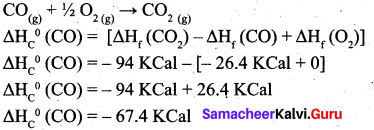Question 8.
In a chemical equilibrium, the rate constant for the forward reaction is 2.5 x 102 and the equilibrium constant is 50. The rate constant for the reverse reaction is
(a) 11.5
(b) 5
(c) 2 x 102
(d) 2 x 10-3
(b) 5

Solution: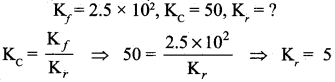Question 9.
The Vant Hoff factor (i) for a dilute aqueous solution of the strong electrolyte barium hydroxide is ………………………………. . [NEET]
(a) O
(b) 1
(c) 2
(d) 3
(b) 1Solution: Ba(OH)2 dissociates to form Ba2 and 20H- ion
$$\alpha=\frac{(i-1)}{(n-1)}$$
i = α (n – 1) + 1
∴ n = i = 3 (for Ba (OH)2, α = 1)

Question 10.
Match the list I and list II using the correct code given below in the list: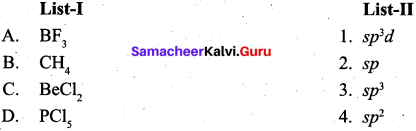Code: A B C D
(a) 4 3 2 1
(b) 1 2 3 4
(c) 3 1 4 2
(d) 2 4 1 3
(a) 4 3 2 1

Question 11.
Nitrogen detection in an organic compound is carried out by Lassaigne’s test. The blue colour formed is due to the formation of
(a) Fe3 [Fe(CN)6]2
(b) Fe4 [Fe(CN)6]3
(c) Fe4 [Fe(CN)6]2
(d) Fe3 [Fe(CN)6]3
(b) Fe4 [Fe(CN)6]3Question 12.
What is the hybridisation state of benzyl carbonium ion?
(a) sp2
(b) spd2
(c) sp2
(d) sp2d
(a) sp2

Question 13.
Statement – I: Boiling point of methane is lower than that of butane.
Statement – II: The boiling point of continuous chain alkanes increases with increase in length of carbon chain.
(a) Statement -I and II are correct and statement – II is correct explanation of statement – I.
(b) Statement – I and II are correct but statement – II is not correct explanation of statement – I.
(c) Statement – I is correct but statement – II is wrong.
(d) Statement – II is wrong but statement – 1 is correct.
(a) Statement -I and II are correct and statement – II is correct explanation of statement – I.Question 14.
In the reaction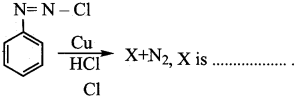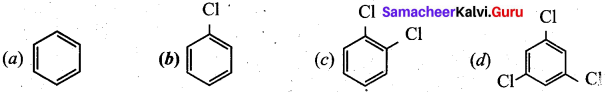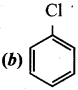Question 15.
Which of the following binds with haemoglobin and reduce the oxygen-carrying capacity of blood?
(a) CO2
(b) NO2
(c) SO3
(d) CO
(d) COPart – II
Answer any six questions in which question No. 21 is compulsory. [6 x 2 = 12]

Question 16.
State and explain Pauli’s exclusion principle.
Pauli’s exclusion principle states that “No two electrons in an atom can have the same set of values of all four quantum numbers”.
Illustration: H(Z = 1) Is1.

One electron is present in hydrogen atom, the four quantum numbers are n – 1, / = 0, m = 0 and s = + ½. For helium Z = 2. He: Is2. In this one electron has the quantum number same as that of hydrogen. n = l, l = 0m = 0 and s = + ½. For other electron, fourth quantum number is different. i.e. n = 1, l = 0, m = 0 and s = – ½.

Question 17.
Give the general electronic configuration of lanthanides and actinides.

• The electronic configuration of lanthanides is 4f1-14 5d10-1 6s2.
• The electronic configuration of actinides is 5f1-14 6d10-1 7s2.Question 18.
Why alkaline earth metals are harder than alkali metals?

1. The strength of metallic bond in alkaline earth metals is higher than alkali metals due to the presence of 2 electrons in its outermost shell as compared to alkali metals, which have only 1 electron in valence shell. Therefore, alkaline earth metals are harder than alkali metals.
2. The alkaline earth metals have greater nuclear charge and more valence electrons, thus metallic bonding is more effective. Due to this they are harder than alkali metals.

Question 19.
What are the limitations of the thermodynamics?

1. Thermodynamics suggests feasibility of reaction but fails to suggest rate of reaction. It is concerned only with the initial and the final states of the system. It is not concerned with the path by which the change occurs.
2. It does not reveal the mechanism of a process.

Question 20.
Write the application of equilibrium constant?

• predict the direction in which the net reaction will take place
• predict the extent of the reaction and
• calculate the equilibrium concentrations of the reactants and products.Question 21.
How much volume of 6 M solution of NaOH is required to prepare 500 ml of 0.250 M NaOH solution?
6% $$\frac{v}{v}$$ aqueous solution contains 6g of methanol in 100 ml solution.

∴ To prepare 500 ml of 6% v/v solution of methanol 30g methanol is taken in a 500 ml standard flask and required quantity of water is added to make up the solution to 500 ml.

Question 22.
Define – Inductive effect.

• It is defined as the change in the polarization of a covalent bond due to the presence of adjacent . bonded atoms or groups in the molecule. It is denoted as I-effect.
• Atoms or groups which lose electron towards a carbon atom are said to have a +1 effect.
Example, CH3 -, (CH3)2 CH (CH3)2 C- etc.
• Atoms or groups which draw electrons away from a carbon atom are said to have a -I effect.
Example, -NO2 -I, -Br, -OH, C6H5 etc.Question 23.
How will you convert n-hexane into benzene? ,
Alkanes with six to ten carbon atoms are converted into homologous of benzene at higher temperature and in the presence of a catalyst. This process is known as aromatisation.

For example, n -Hexane passed over Cr2O3 supported on alumina at 873 K gives benzene.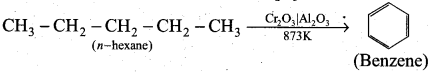Question 24.
Why haloalkanes have higher boiling point than parent alkanes having the same number of carbons?
Haloalkanes have higher boiling point than the parent alkane having the same number of carbon atoms because the intermolecular forces of attraction and dipole-dipole interactions are comparatively stronger in haloalkanes.

Part-III

Answer any six questions in which question No. 25 is compulsory. [6 x 3 = 18]Question 25.
Balance the following equations by using oxidation number method.
K2Cr2O7 + KI + H2SO4 → K2SO4 + Cr2(SO4)3 + I2 + H2O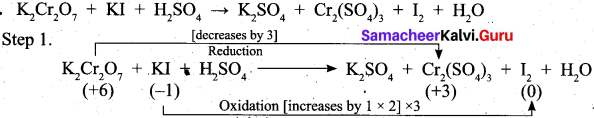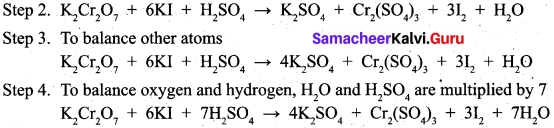Question 26.
Explain the periodic trend of ionization potential.
(a) The energy required to remove the most loosely held electron from an isolated gaseous atom is called as ionization energy.
(b) Variation in a period.

Ionization energy is a periodic property.
On moving across a period from left to right, the ionization enthalpy value increases. This is due to the following reasons.

• Increase of nuclear charge in a period
• Decrease of atomic size in a periodBecause of these reasons, the valence electrons are held more tightly by the nucleus. Therefore, ionization enthalpy increases.

(c) Variation in a group
As we move from top to bottom along a group, the ionization enthalpy decreases. This is due to the following reasons.

• A gradual increase in atomic size
• Increase of screening effect on the outermost electrons due to the increase of number of inner electrons.

Hence, ionization enthalpy is a periodic property.

Question 27.
Distinguish between hard water and soft water.

 Hard water Soft water Presence of magnesium and calcium in the form of bicarbonate, chloride and sulphate in water makes hard water. Presence of soluble salts of calcium and magnesium in water makes it soft water. Cleaning capacity of soap is reduced when used in hard water. Cleaning capacity of soap is more when used in soft water. When hard water is boiled deposits of insoluble carbonates of magnesium and calcium are obtained. When soft water is boiled, there is no deposition of salts.

Question 28.
Explain how the equilibrium constant Kc predict the extent of a reaction.
(i) The value of equilibrium constant Kc tells us the extent of the reaction i.e., it indicates how far the reaction has proceeded towards product formation at a given temperature.
(ii) A large value of Kc indicates that the reaction reaches equilibrium with high product yield on the other hand, lower value of Kc indicates that the reaction reaches equilibrium with low product yield.
(iii) If Kc > 103, the reaction proceeds nearly to completion.
(iv) If Kc < 10-3 the reaction rarely proceeds.
(v) It the Kc is in the range 10-3 to 103, a significant amount of both reactants and products are present at equilibrium.Question 29.
How many moles of solute particles are present in one litre of 10-4 M K2SO4?
In 10-4 M K2 SC4), solution, there are 10-4 moles of potassium sulphate.
K2SO4 molecule contains 3 ions (2K+ and 1 SO42-)
1 m;ole of I^SO,, contains 3 x 6.023 x 1023 ions
10″4 mole of K2SO4 contains 3 x 6.023 x 1023 x 10-4 ions = 18.069 x 1019

Question 30.
Draw the Lewis structures for the following.
(i) SO42-
(ii) O3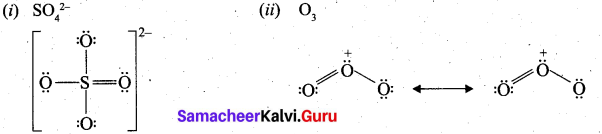Question 31.
Write a short notes on hyperconjugation.

• The delocalization of electrons of a bond is called as hyperconjugation. It is a special stabilizing effect that results due to the interaction of electrons of a cr bond with the adjacent empty non-bonding P-orbitals resulting in an extended molecular orbital.
• Hyperconjugation is a permanent effect.
• For example, in propene, the a- electrons of C—H bond of methyl group can be delocalised into the n- orbital of doubly bonded carbon as represented below.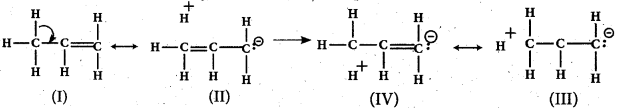Question 32.
How will you prepare propyne using alkyene dihalide?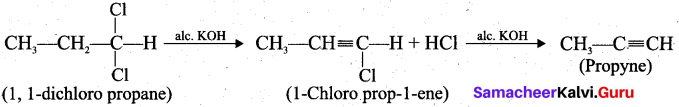Question 33.
Explain how the oxides of sulphur pollute the atomospheric air? Give its harmful effects.

• Sulphur dioxide and sulphur trioxide are produced by burning sulphur-containing fossil fuels and by roasting of sulphide ores.
• SO2 is a poisonous gas for both animals and plants. SO2 causes eye irritation, coughing and respiratory diseases like asthma, bronchitis.
• SO2 is oxidised to more harmful SO3 gas in the presence of particulate matter present in the polluted air:
SO3 combines with atmospheric water vapour to form H2SO4 which comes down along with rain in the form of acid rain:
• Acid rain causes stone leprosy, affect aquatic ecosystem, corrode water pipes and causes respiratory ailment in humans and animals.Part – IV

Question 34.
(a) Urea is prepared by the reaction between ammonia and arbondioxide.
2NH3(g) + CO2(g) → (NH2)2 CO(aq) + H2O(l)
In one process, 637.2 g of NH3 are allowed to react with 1142 g of CO2.
(i) Which of the two reactants is the limiting reagent? (2)
(ii) Calculate the mass of (NH2)2 CO formed. (2)
(iii) How much Of the excess reagent in grams is left at the end of the reaction? (1)
[OR]
(b) (i) A student reported the ionic radii of isoelectronic species X3+, Y2+ and Z as 136 pm, 64 pm and 49 pm respectively. Is that order correct? Comment. (2)
(ii) Explain any three factors which influence the ionization energy. (3)
(a) (i) 2NH3(g) + CO2(g) → (NH4)2 CO(aq) + H2O(l)
2 moles 1 mole 1 mole
No. of moles of ammonia =$$\frac{637.2}{17}$$ = 37.45 mole
No. of moles of CO2 = $$\frac{1142}{44}$$ = 25.95 mole

As per the balanced equation, one mole of C02 requires 2 moles of ammonia.
∴ No. of moles of NH3 required to react with 25.95 moles of CO2 is
= $$\frac{2}{1}$$ x 25.95 = 51.90 moles.
∴ 37.45 moles of NH3 is not enough to completely react with CO2 (25.95 moles).
Hence, NH3 must be the limiting reagent, and CO2 is excess reagent.
(ii) 2 moles of ammonia produce 1 mole of urea.
∴ Limiting reagent 37.45 moles of NH3 can produce $$\frac{1}{2}$$ x 37.45 moles of urea.
= 18.725 moles of urea.
∴ The mass of 18.725 moles of urea = No. of moles x Molar mass
= 18.725 x 60
= 1123.5 g of urea.(iii) 2 moles of ammonia requires 1 mole of CO2.
∴ Limiting reagent 37.45 moles of NH3 will require $$\frac{1}{2}$$ x 37.45 moles of CO2.
= 18.725 moles of CO2.
∴ No. of moles of the excess reagent (CO2) left = 25.95 – 18.725 = 7.225
The mass of the excess reagent (C02) left = 7.225 x 44
= 317.9 g of CO2.
[OR]

(b) (i) X3+, Y2+, Z are isoelectronic.
∴ Effective nuclear charge is in the order
$$\left(Z_{\text {eff }}\right)_{Z}<\left(Z_{\text {eff }}\right)_{Y^{2+}}<\left(Z_{\text {eff }}\right)_{X^{3+}}$$ and hence, ionic radii should be in the order $$r_{z}>r_{y^{2}}>r_{x^{3+}}$$
∴ The correct values are:

 Species Ionic radii Z 136 Y2+ 64 X3+ 49

(ii) 1. Size of the atom: If the size of an atom is larger, the outermost electron shell from the nucleus is also larger and hence the outermost electrons experience lesser force of attraction. Hence it would be more easy to remove an electron from the outermost shell. Thus, ionization energy decreases with increasing atomic sizes.
$$Ionization enthalpy \propto \frac{1}{\text { Atomic size }}$$

2. Magnitude of nuclear charge: As the nuclear charge increases, the force of attraction . between the nucleus and valence electrons also increases. So, more energy is required to remove a valence electron. Hence I.E increases with increase in nuclear charge.
Ionization enthalpy α nuclear charge3. Screening or shielding effect of the inner electrons: The electrons of inner shells form a cloud of negative charge and this shields the outer electron from the nucleus. This screen reduces the coulombic attraction between the positive nucleus and the negative outer electrons. If screening effect increases, ionization energy decreases.
$$Ionization enthalpy \propto \frac{1}{\text { Screening effects }}$$

4. Penetrating power of subshells s, p, d and f: The s-orbital penetrate more closely to the nucleus as compared to p-orbitals. Thus, electrons in s-orbitals are more tightly held by the nucleus than electrons in p-orbitals. Due to this, more energy is required to remove a electron from an s-orbital as compared to a p-orbital. For the same value of ‘n’, the penetration power decreases in a given shell in the order, s > p > d > f.5. Electronic configuration: If the atoms of elements have either completely filled or exactly half filled electronic configuration, then the ionization energy increases.

Question 35.
(a) (i) Write the important common features of group 2 elements? (3)
(ii) What is meant by retrograde solubility? (2)
[OR]
(b) (i) Derive a general expression for the equilibrium constant Kp and Kc for the reaction. (3)
(ii) Write the Kp and Kc for NH3 formation reaction. (2)
(a) (i)

• Group 2 elements except beryllium are commonly known as alkaline earth metals because their oxides and hydroxides are alkaline in nature and these metal oxides are found in the Earth’s crust.
• Many alkaline earth metals are used in creating colours and used in fireworks.
• Their general electronic configuration is ns2.
• Atomic and ionic radii of alkaline earth metals are smaller than alkali metals, on moving down the group, the radii increases.
• These elements exhibit +2 oxidation state in their compounds.
• Alkaline earth metals have higher ionizatoin enthalpy values than alkali metals and they are less electropositive than alkali metals.
• Hydration enthalpies of alkaline earth metals decreases as we go down the group.
• Electronegativity values of alkaline earth metals decrease down the group.
• Alkaline earth metal salts moistened with concentrated hydrochloric acid gave a characteristic coloured flame, when heated on a platinum wire in a flame.(ii) Gypsum is a soft mineral and it is less soluble in water as the temperature increases. This is known as retrograde solubility, which is a distinguishing characteristic of gypsum.

[OR]

(b) (i) Consider a general reaction in which all reactants and products are ideal gases.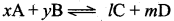The equilibrium constant kc is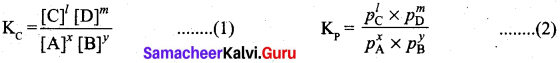The ideal gas equation is PV = nRT or P = $$\frac{n}{V}$$ RT Since, Active mass = molar concentration = $$\frac{n}{V}$$
p = Active mass x RT

Based on the above expression, the partial pressure of the reacants and products can be expressed as,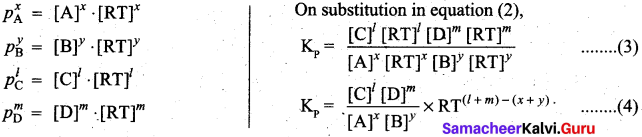By comparing equation (1) and (4), we get Kp = Kc (RT)(Δng) ………………………. (5)

where Δng is the difference between the sum of number of moles of products and the sum of number of moles of reactants in the gas phase.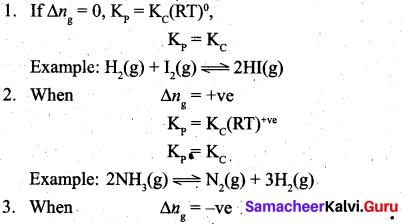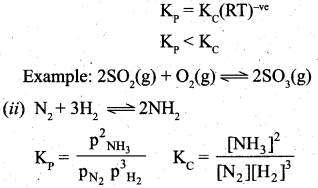Question 36.
(a) (i) 0.24 g of a gas dissolves in 1 L of water at 1.5 atm pressure. Calculate the amount of dissolved gas when the pressure is raised to 6.0 atm at constant temperature. (3) (ii) What is a vapour pressure of liquid? (2)

[OR]

(b) (i) Explain the ionic bond formation in MgO and CaF2. (3)
(ii) What is bond angle?
(a) (i) $$P_{\text {solute }}=\mathrm{K}_{\mathrm{H}} x_{\text {solute in solution }}$$
At pressure 1.5 atm, $$p_{1}=\mathbf{K}_{\mathrm{H}} x_{1}$$  ……………. (1)
At pressure 6.0 atm, $$p_{2}=\mathbf{K}_{\mathbf{H}} \boldsymbol{x}_{\mathbf{2}}$$ ………………… (2)
Dividing equation (1) by (2)
We get $$\frac{p_{1}}{p_{2}}=\frac{x_{1}}{x_{2}} \Rightarrow \frac{1.5}{6.0}=\frac{0.24}{x_{2}}$$
Therefore $$x_{2}=\frac{0.24 \times 6.0}{1.5}=0.96 \mathrm{g} / \mathrm{L}$$(ii) 1. The pressure of the vapour in equilibrium with its liquid is called vapour pressure of the liquid at the given temperature.
2. The relative lowering of vapour pressure is defined as the ratio of lowering of vapour pressure to vapour pressure of pure solvent.
Relative lowering of vapour pressure = $$\frac{p_{\text {solvent }}^{\circ}-p_{\text {solution }}}{p_{\text {solvent }}^{\circ}}$$

[OR]

(b) (i) Magnesium oxide (MgO) :
Electronic configuration of Mg – ls2 2s2 2p5 3s2
Electronic configuration of O – ls2 2s2 2p6 3s2 3p4

• Magnesium has two electrons in its valence shell and oxygen has six electrons in its valence shell.
• By losing two electrons, Mg acquires the inert gas configuration of Neon and becomes a dipositive cation, Mg2+:
$$\mathrm{Mg} \longrightarrow \mathrm{Mg}^{2+}+2 e^{-}$$
• Oxygen accepts the two electrons to become a dinegative oxide anion, O2- thereby attaining the inert gas configuration of Neon:
$$0+2 e^{-} \longrightarrow 0^{2-}$$
• These two ions, Mg2+ and O2- combine to form an ionic crystal in which they are held together by electrostatic attractive forces.
• During the formation of magnesium oxide crystal 601.6 kJ mol-1 energy is released. This favours the formation of magnesium oxide (MgO) and its stabilisation.CaF2, Calcium fluoride:

• Calcium, Ca : [Ar] 4s2 Fluorine, F : [He] 2s2 2p5
• Calcium has two electrons in its valence shell and fluorine has seven electrons in its valence shell.
• By losing two electrons, calcium attains the inert gas configuration of Argon and becomes a dipositive cation, Ca2+.
• Two fluorine atoms, each one accepts one electron to become two uninegative fluoride ions (F-) thereby attaining the stable configuration of Neon.
• These three ions combine to form an ionic crystal in which they are held together by electrostatic attractive force.
• During the formation of calcium fluoride crystal 1225.91 kJ mol-1 of energy is released. This favours the formation of calcium fluoride, CaF2 and its stabilisation.

(ii) Covalent bonds are directional in nature and are oriented in specific direction in space. This directional nature creates a fixed angle between two covalent bonds in a molecule and this angle is termed as bond angle.

Question 37.
(a) (i) 0.33 g of an organic compound containing phosphorous gave 0.397 g of Mg2P2O7 by the analysis. Calculate the percentage of P in the compound. (3)
(ii) Give the IUPAC names of the following compounds. (2)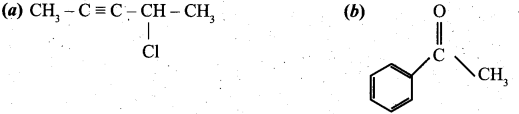[OR]

(b) (i) Describe the mechanism of nitration of benzene. (3)
(ii) How will you prepare m-dinitro benzene. (2)
(a) (i) Weight of organic substance (w) = 0.33g
Weight of Mg2P2O7 (x) = 0.397g
Percentage of phosphorous = $$\frac{62}{222} \times \frac{x}{w} \times 100=\frac{62}{222} \times \frac{0.397}{0.33} \times 100=33.59 \%$$
(ii) (a) 4-chloropent-2-ype .
(b) Acetophenone(b) (i) Step-1: Generation of NO2 electrophile.
HNO3 + H2SO4 → NO2 + HSO4 + H2O

Step-2: Attack of the electrophile on benzene ring to form arenium ion.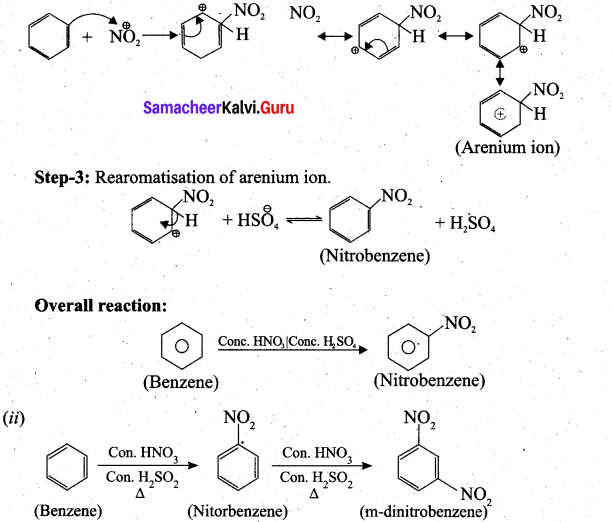Question 38.
(a) Complete the following reaction, identify the products.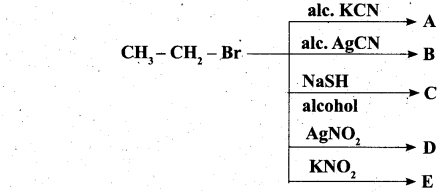[OR]
(b) (i) What is green chemistry?
(ii) Differentiate – BOD and COD.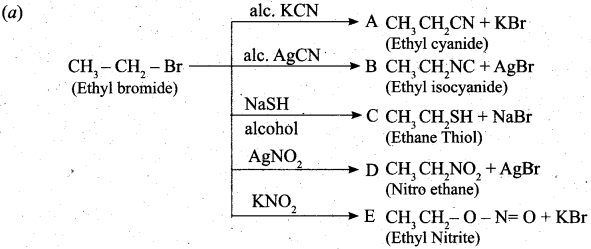[OR]
(b) (i)

• Green chemistry is a chemical philosophy encouraging the design of products and processes that reduces or eliminates the use and generation of hazardous substances.
• Efforts to control environmental pollution resulted in the development of science for the synthesis of chemicals favourable to the environment.
• Green chemistry means the science of environmentally favourable chemical synthesis.(ii)

 Biochemical oxygen demand (BOD) Chemical oxygen demand (COD) The total amount of oxygen (in milligrams) consumed by microorganisms in decomposing the waste in one litre of water at 20°C for a period of 5 days is called biochemical oxygen demand (BOD). Chemical oxygen demand is defined as the amount of oxygen required by the organic matter in a sample of water for its oxidation by a strong oxidising agent like K2Cr2O7 in acidic medium for a period of 2 hours. Its value is expressed in ppm. Its value is expressed in mg/litre. BOD is used as a measure of degree of water pollution. COD is a measure of amount of organic compounds in a water sample. BOD is only a measurement of consumed oxygen by microorganims to decompose the organic matter. COD refers to the requirement of dissolved oxygen for both the oxidation of organic and inorganic constituents. Clean water would have BOD value less than 5 ppm. Clean water would have COD value greater than 250 mg/litre.